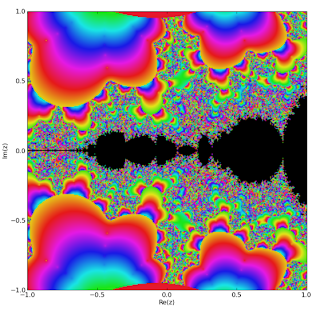# Jacobi theta function fractals

January 15, 2009

gaspaheangea notified me of a fractal rendering made by modifying my mandelbrot.py script to iterate a Jacobi theta function instead of the standard quadratic function. The result is quite gorgeous.

I’ve plotted a slightly variation of the same fractal, with q = 0.5 (instead of about 0.04):By trying different parameters, still more interesting views can be found.

With 100K points, the above image took about 20 minutes to plot. Arbitrary-precision arithmetic is really unnecessary for this (except at high zoom); the Jacobi theta functions can be implemented quite easily with ordinary floating-point arithmetic, and would be orders of magnitude faster as such. So if anyone reading this is looking for a fun math-related mini-project to work on, there’s an idea.